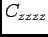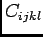# OOF: Finite Element Analysis of Microstructures

damageNext: griffith and griffith2 Up: Element Types and their Previous: damisotropic   Contents

Subsections

## damage

This is an isotropic element with more control over the way damage is incurred. See Section 4.5.9 for discussion on mutating elements and damage.

The idea is that the stiffness drop in the direction of maximum tension may be different than the stiffness drop associated with other directions. For instance, if a brick develops a crack it can still support loads in directions which are not normal to the crack plane.

There is one user-defined upper bound and two user-defined knockdown factors.

The process of mutation is the following:

1. The maximum principal stress (eigenvalue) is calculated and compared to the specified maximum stress.
2. If the maximum specified stress is exceeded, the direction of the principal stress axis is determined. (This direction may be out of the plane.) The stiffness matrix is rotated into a new coordinate system in which the principal stress lies along the z axis.
3. In the rotated coordinate system,is multiplied by knockdown1. All other components ofwith at least one of ijkl=z are multiplied by knockdown2.
4. The stiffness matrix is rotated back into its original orientation.

### Parameters

orientation
The orientation must be specified, but it has no effect since the thermoelastic coefficients are isotropic before mutation. The orientation is calculated in the mutation step, but the element is rotated and then rotated back, so the orientation after mutation contains no useful information either.4.5 The orientation of the principal stress axis is reported in the message window as elements mutate. [degrees]

thermoelastic coefficients
All thermoelastic coefficients for a undamaged damage element are isotropic as described in section 4.5.1.

maxstress
The value to which the maximum principal stress is compared to see if the element should incur damage when the mutate command is invoked. See comments in section 4.5.9. [stress]

knockdown1
The factor which multiplies the rotated stiffness matrix'scomponent during a mutation. [dimensionless]

knockdown2
The factor which multiplies the rotated stiffness matrix's remainingcomponents for at least one of ijkl=z during a mutation. [dimensionless]

only_once
If true, an element which has been damaged during one mutate command will not be damaged further on subsequent mutate commands. If false, the knockdown factors may be applied multiple times. Default: falseNext: griffith and griffith2 Up: Element Types and their Previous: damisotropic   Contents
/* Send mail to the OOF Team *//* Go to the OOF Home Page */